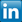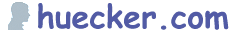GoTo: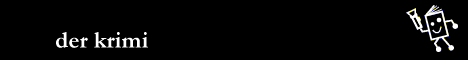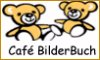Lesezeichen [ Info # Jobs # QR-Code # Haikus ] Di 28 März 2023 06:57:16

 huecker.com # Grundlagen der Programmierung | Tcl Tutorial. -- . More Examples Of Regular Expressions . Regular expressions provide a very powerful method of defining a pattern, but they are a bit awkward to understand and to use properly. So let us examine some more examples in detail. We start with a simple yet non-trivial example: finding floating-point numbers in a line of text. Do not worry: we will keep the problem simpler than it is in its full generality. We only consider numbers like 1.0 and not 1.00e+01. How do we design our regular expression for this problem? By examining typical examples of the strings we want to match: Valid numbers are: 1.0, .02, +0., 1, +1, -0.0120 Invalid numbers (that is, strings we do not want to recognise as numbers but superficially look like them): -, +., 0.0.1, 0..2, ++1 Questionable numbers are: +0000 and 0001 We will accept them - because they normally are accepted and because excluding them makes our pattern more complicated. A pattern is beginning to emerge: A number can start with a sign (- or +) or with a digit. This can be captured with the expression [-+]?, which matches a single "-", a single "+" or nothing. A number can have zero or more digits in front of a single period (.) and it can have zero or more digits following the period. Perhaps: [0-9]*\.[0-9]* will do ... A number may not contain a period at all. So, revise the previous expression to: [0-9]*\.?[0-9]* The total expression is: [-+]?[0-9]*\.?[0-9]* At this point we can do three things: Try the expression with a bunch of examples like the ones above and see if the proper ones match and the others do not. Try to make it look nicer, before we start off testing it. For instance the class of characters "[0-9]" is so common that it has a shortcut, "\d". So, we could settle for: [-+]?\d*\.?\d* instead. Or we could decide that we want to capture the digits before and after the period for special processing: [-+]?([0-9])*\.?([0-9]*) Or, and that may be a good strategy in general!, we can carefully examine the pattern before we start actually using it. You see, there is a problem with the above pattern: all the parts are optional, that is, each part can match a null string - no sign, no digits before the period, no period, no digits after the period. In other words: Our pattern can match an empty string! Our questionable numbers, like "+000" will be perfectly acceptable and we (grudgingly) agree. But more surprisingly, the strings "--1" and "A1B2" will be accepted too! Why? Because the pattern can start anywhere in the string, so it would match the substrings "-1" and "1" respectively! We need to reconsider our pattern - it is too simple, too permissive: The character before a minus or a plus, if there is any, can not be another digit, a period or a minus or plus. Let us make it a space or a tab or the beginning of the string: ^|[ \t] - This may look a bit strange, but what it says is: either the beginning of the string (^ outside the square brackets) - or (the vertical bar) a space or tab (remember: the string "\t" represents the tab character). Any sequence of digits before the period (if there is one) is allowed: [0-9]+\.? There may be zero digits in front of the period, but then there must be at least one digit behind it: \.[0-9]+ And of course digits in front and behind the period: [0-9]+\.[0-9]+ The character after the string (if any) can not be a "+","-" or "." as that would get us into the unacceptable number-like strings: \$|[^+-.] (The dollar sign signifies the end of the string). Before trying to write down the complete regular expression, let us see what different forms we have: No period: [-+]?[0-9]+ A period without digits before it: [-+]?\.[0-9]+ Digits before a period, and possibly digits after it: [-+]?[0-9]+\.[0-9]* Now the synthesis: (^|[ \t])([-+]?([0-9]+|\.[0-9]+|[0-9]+\.[0-9]*))(\$|[^+-.]) Or: (^|[ \t])([-+]?(\d+|\.\d+|\d+\.\d*))(\$|[^+-.]) The parentheses are needed to distinguish the alternatives introduced by the vertical bar and to capture the substring we want to have. Each set of parentheses also defines a substring and this can be put into a separate variable: regexp {.....} \$line whole char_before number nosign char_after # Or simply only the recognised number (x's as placeholders), the # last can be left out. regexp {.....} \$line x x number Tip: To identify these substrings: just count the opening parentheses from left to right. If we put it to the test: ``` set pattern {(^|[ \t])([-+]?(\d+|\.\d+|\d+\.\d*))(\$|[^+-.])} set examples {"1.0" " .02" " +0." "1" "+1" " -0.0120" "+0000" " - " "+." "0001" "0..2" "++1" "A1.0B" "A1"} foreach e \$examples { if { [regexp \$pattern \$e whole\ char_before number digits_before_period] } { puts ">>\$e<<: \$number (\$whole)" } else { puts ">>\$e<<: Does not contain a valid number" } } ``` the result is: ``` >>1.0<<: 1.0 (1.0) >> .02<<: .02 ( .02) >> +0.<<: +0. ( +0.) >>1<<: 1 (1) >>+1<<: +1 (+1) >> -0.0120<<: -0.0120 ( -0.0120) >>+0000<<: +0000 (+0000) >> - <<: Does not contain a valid number >>+.<<: Does not contain a valid number >>0001<<: 0001 (0001) >>0..2<<: Does not contain a valid number >>++1<<: Does not contain a valid number >>A1.0B<<: Does not contain a valid number >>A1<<: Does not contain a valid number ``` So our pattern correctly accepts the strings we intended to be recognised as numbers and rejects the others. Let us turn to some other patterns now: Text enclosed in a string: This is "quoted text". If we know the enclosing character in advance (double quotes, " in this case), then "([^"])*" will capture the text inside the double quotes. Suppose we do not know the enclosing character (it can be " or '). Then: regexp {(["'])[^"']*\1} \$string enclosed_string will do it - the \1 is a so-called back-reference to the first captured substring. You can use this technique to see if a word occurs twice in the same line of text: ``` set string "Again and again and again ..." if { [regexp {(\y\w+\y).+\1} \$string => word] } { puts "The word \$word occurs at least twice" } ``` (The pattern \y matches the beginning or the end of a word and \w+ indicates we want at least one character.) Suppose you need to check the parentheses in some mathematical expression: (1+a)/(1-b*x) for instance. A simple check is counting the open and close parentheses: ``` # Use the return value of [regexp] to count the number of # parentheses ... set string "(Again and again and again ..." if { [regexp -all {\(} \$string] != [regexp -all {\)} \$string] } { puts "Parentheses unbalanced!" } ``` Of course, this is just a rough check. A better one is to see if at any point while scanning the string there are more close parentheses than open parentheses. We can easily extract the parentheses and put them in a list (the -inline option does that): ``` set parens [regexp -inline -all {[()]} \$string] set balance 0 set change("(") 1 ;# This technique saves an if-block :) set change(")") -1 foreach p \$parens { incr balance \$change(\$p) if { \$balance < 0 } { puts "Parentheses unbalanced!" } } ``` Finally: Regular expressions are very powerful, but they have certain theoretical limitations. One of these limitations is that they are not suitable for parsing arbitrarily nested text. You can experiment with regular expressions using the VisualRegexp or Visual REGEXP applications. More on the theoretical background and practical use of regular expressions (there is lots to cover!) can be found in the book Mastering Regular Expressions by J. Friedl.
 --[ Home | Top ] [ . Previous | Index | Next . ]
Der Inhalt dieser Seite wurde am 06.11.2019 um 12.19 Uhr aktualisiert.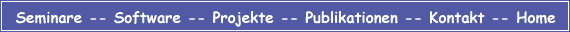huecker dot com * Germany | Datenschutz © 1999, 2023 Franz-Josef Hücker. All Rights Reserved.